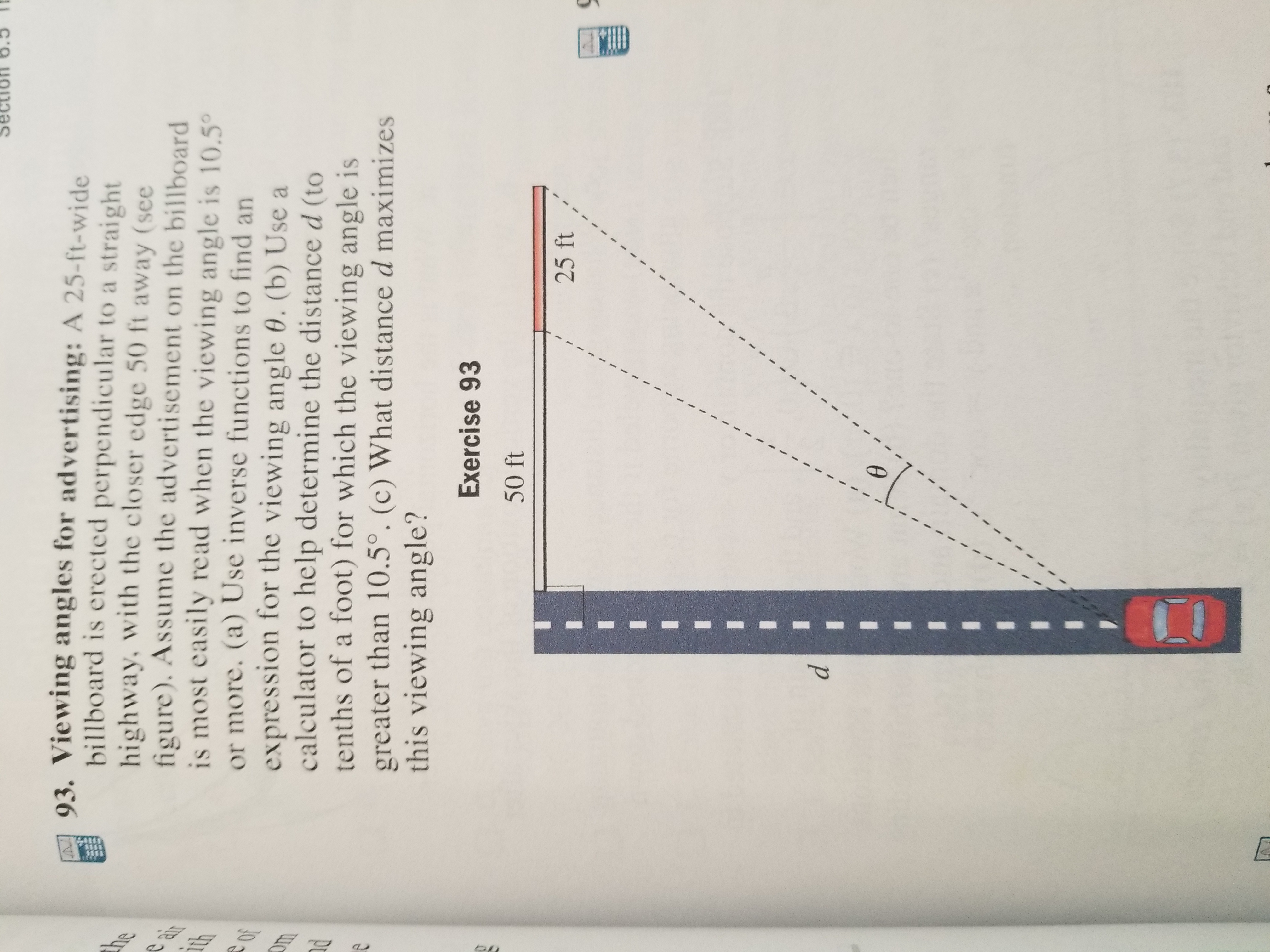# 93. Viewing angles for advertising: A 25-ft-widebillboard is erected perpendicular to a strai ghthighway, with the closer edge 50 ft away (seefigure). Assume the adverti sement on the billboardis most easily read when the viewing angle is 10.5°or more. (a) Use inverse functions to find anexpression for the viewing angle 0. (b) Use acalculator to help determine the distance d (totenths of a foot) for which the viewing angle isgreater than 10.5°. (c) What distance d maximizesthis viewing angle?nemleExercise 9350 ft25 ftair

Question
9 viewshelp_outlineImage Transcriptionclose93. Viewing angles for advertising: A 25-ft-wide billboard is erected perpendicular to a strai ght highway, with the closer edge 50 ft away (see figure). Assume the adverti sement on the billboard is most easily read when the viewing angle is 10.5° or more. (a) Use inverse functions to find an expression for the viewing angle 0. (b) Use a calculator to help determine the distance d (to tenths of a foot) for which the viewing angle is greater than 10.5°. (c) What distance d maximizes this viewing angle? ne m le Exercise 93 50 ft 25 ft air fullscreen
check_circle

Step 1

To determine:

Part a) Expression for the viewing angle.

Part b) The distance d for which the viewing angle is greater than 10.50.

Part c) The distance d for which the maximize the viewing angle.

Step 2

Given:

Step 3

Formula Used:

...

### Want to see the full answer?

See Solution

#### Want to see this answer and more?

Solutions are written by subject experts who are available 24/7. Questions are typically answered within 1 hour.*

See Solution
*Response times may vary by subject and question.
Tagged in

### Calculus